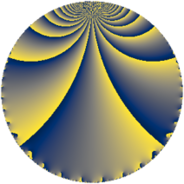# Properties

 Label 1216.4.tLevel $1216$ Weight $4$ Character orbit 1216.t Rep. character $\chi_{1216}(353,\cdot)$ Character field $\Q(\zeta_{6})$ Dimension $240$ Sturm bound $640$

# Related objects

## Defining parameters

 Level: $$N$$ $$=$$ $$1216 = 2^{6} \cdot 19$$ Weight: $$k$$ $$=$$ $$4$$ Character orbit: $$[\chi]$$ $$=$$ 1216.t (of order $$6$$ and degree $$2$$) Character conductor: $$\operatorname{cond}(\chi)$$ $$=$$ $$152$$ Character field: $$\Q(\zeta_{6})$$ Sturm bound: $$640$$

## Dimensions

The following table gives the dimensions of various subspaces of $$M_{4}(1216, [\chi])$$.

Total New Old
Modular forms 984 240 744
Cusp forms 936 240 696
Eisenstein series 48 0 48

## Trace form

 $$240q + 1080q^{9} + O(q^{10})$$ $$240q + 1080q^{9} + 3000q^{25} + 1392q^{33} + 120q^{41} + 10320q^{49} + 1368q^{57} + 432q^{73} - 6960q^{81} + 3168q^{97} + O(q^{100})$$

## Decomposition of $$S_{4}^{\mathrm{new}}(1216, [\chi])$$ into newform subspaces

The newforms in this space have not yet been added to the LMFDB.

## Decomposition of $$S_{4}^{\mathrm{old}}(1216, [\chi])$$ into lower level spaces

$$S_{4}^{\mathrm{old}}(1216, [\chi]) \cong$$ $$S_{4}^{\mathrm{new}}(152, [\chi])$$$$^{\oplus 4}$$$$\oplus$$$$S_{4}^{\mathrm{new}}(608, [\chi])$$$$^{\oplus 2}$$